# THE USER S INFERENCE MODEL

## THE USER'S INFERENCE MODEL

Suppose that our user is interested in the value of a sensitive query Q, which henceforth will be referred to as the target-query. If he asks Q, the query-answering system of the statistical database will refuse to answer Q. But, as shown in the previous examples, the user could exactly compute or estimate the value of Q from the values of his (non-sensitive) queries that have been previously answered. We now introduce a formal method which allows the user to decide if the target-query is indirectly answered in an exact or approximate way given the set of previously answered queries. In what follows, we limit our considerations to the case that all queries posed by the user are either count queries or sum queries sharing the summary attribute, so that queries are fully specified by their category predicates; moreover, we assume that the values of each descriptive attribute are exhaustive and mutually exclusive. By b = (b, b′′,) we denote the multidimensional attribute whose components are the descriptive attributes of the records in the database; we shall refer to b as the category variable. Let B be the domain of b; that is, B is the Cartesian product of the domains of b, b′′,. A query is called disjunctive if its category predicate is given by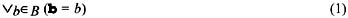where B is a nonempty subset of B; such a query will be denoted by Q(B). Note that the number of possible distinct disjunctive queries is 2|B|1. Owing to the exhaustive and mutually exclusive nature of values of the descriptive attributes, every nonnull query is equivalent to a disjunctive query (Malvestuto & Moscarini, 1990) since its category predicate is logically equivalent to a formula such as (1). Therefore, without loss of generality, we shall consider disjunctive queries only, and in what follows the adjective "disjunctive" is omitted.

Suppose that, before asking the target-query, the user posed n queries Q1,, Qn which were all answered. Let Q = {Q1,, Qn}, let Qv = Q(Bv) where Bv is a nonempty subset of B, and let qv be the value of Qv, 1 v n. The set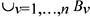will be referred to as the active domain of the category variable (with respect to Q). By the characteristic partition of the active domain of b, we mean its coarsest partition γ = {C1,, Cm} such that the sets Bv belong to the set field generated by γ, that is, each Bv can be obtained as the union of one or more classes of γ. Note that m is not greater than the size of the active domain of b. Let V = {1,, n} and E = {e1,, em} where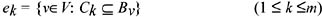The sets V and E will be respectively viewed as being the vertex set and the edge set of a hypergraph H = (V, E), which will be referred to as the hypergraph associated with Q. Note that, if each edge of H contains less than three vertices, then H can be pictured as an ordinary graph and the query set Q is called graphical.

Example 2 (continued): Consider again the three sum queries Q1, Q2, and Q3, and let Q = {Q1, Q2, Q3}. Then, with our notation we have

B3 = {Direction,Services}.

The active domain of department is {Direction, Administration, Services}, and its characteristic partition is the point partition; that is, γ = {C1, C2, C3} where

C1 = {Direction}

C1 = {Services}.

Thus, V = {1, 2, 3} and E = {e1, e2, e3} with e1 = {1, 3}, e2 = {1, 2}, and e3 = {2, 3}. The hypergraph associated with Q is the graph shown in Figure 1 where, for each vertex v, the value of Qv is also reported.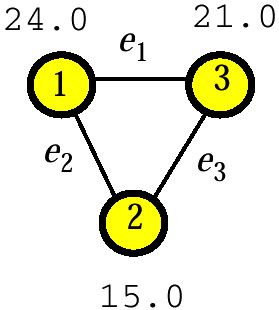Figure 1: The Graph Associated with Three Queries

Let D be the data type of the values qv. Thus, if the queries Qv are count queries, then D is the set Z0+ of nonnegative integers, and if the queries Qv are sum queries, then D is the domain of the summary attribute (typically, D is the set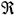of real numbers, or the set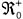of nonnegative real numbers, or the set Z0+ of nonnegative integers). The constraint system associated with Q is the equation system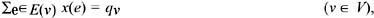where E(v) is the set of edges of H containing vertex v, with the addition of the m type constraints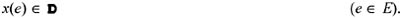Note that, if H = (hv)v V is the incidence matrix of the hypergraph H and q = (qv)v V, then the equation system above can be written in matrix notation as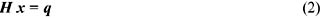The constraint system associated with Q can be summarized in the vertex-weighted hypergraph (H, q), which will be referred to as the answer map of Q. We shall show how it allows the user to decide if the target-query Q is indirectly answered given Q, by which we mean that either the value of Q can be exactly computed from Q or the range of the feasible values of Q given Q is bounded. Before giving the decision algorithms for these two cases, we need to introduce some further definitions.

Given a nonempty set F of edges of H, let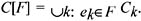By the query corresponding to F, we mean the query Q(C[F]), that is, the query with category predicate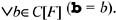Note that, if F = E(v) for some vertex v of H, then C[F] = Bv and the query corresponding to F is exactly Qv. Moreover, if F is a singleton (that is, if F contains exactly one edge), then the query corresponding to F is called an elementary query.

The closure of Q is the set of the queries of the type Q(C[F]) such that the sum expression e F x(e) is a D-invariant in the answer map (H, q), by which we mean that, for every two solutions x1 and x2 of the constraint system associated with Q, one has e F x1(e) = e F x2(e). Thus, if the target-query Q belongs to the closure of Q, then the value of Q can be exactly computed from Q, that is, Q is implicitly answered given Q.

Example 2 (continued): Assume that the domain of salary is. Then, the system of linear constraints associated with Q reads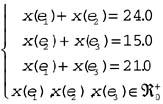Since there is exactly one solution with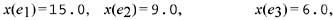every sum query on salary whose category predicate contains only values of department from {Direction, Administration, Services} belongs to the closure of Q. It follows that the sensitive query Q4 is implicitly answered given Q.

Consider now the case that the target-query Q does not belong to the closure of Q but is covered by Q in the sense that there exists a nonempty set F of edges of H such that Q = Q(C[F]). Then the user can determine the tightest lower bound, say α1, and the tightest upper bound, say α2, on the value of Q by solving the following two problems: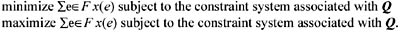If α1 −∞ and α2 +, then the target-query Q is indirectly answered in an approximate way and the intersection of D with the real interval [α1, α2] is called the feasibility range for the value of Q.

Example 3: Let Q = {Q1, Q2, Q3} be a set of additive queries with values from D whose answer map is the vertex-weighted graph shown in Figure 2.Figure 2

Thus, the equation system in the constraint system associated with Q contains the following three equations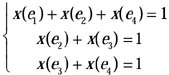and the parametric expression of the general solution of this equation system is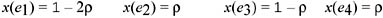Consider now the type constraints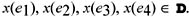We now separately discuss the three cases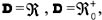and D = Z0+.

If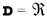, then the parameter ρ ranges from −∞ to +. In this case, it is easy to see that the closure of Q only contains the queries corresponding to the edge sets E(1), E(2), and E(3) and, hence, it coincides with Q. Moreover, for any other nonempty edge set, the tightest lower and upper bounds on the value of the corresponding query are −∞ to +, respectively.

If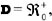then the parameter ρ ranges from 0 to 1/2. Again, the closure of Q coincides with Q. But, for each nonempty edge set F, the feasibility range for the value of the query corresponding to F is bounded. For example, the feasibility range for the value of the query corresponding to F = {e1, e3} is the real interval [1/2, 2]. To sum up, every query covered by Q and not in Q is indirectly answered in an approximate way.

If D = Z0+, then ρ = 0 and, hence, the closure of Q coincides with the set of queries covered by Q.

In the rest of this section, we discuss the computational aspects of the problem of testing the membership of the target-query Q in the closure of Q. Let Q = Q(B) where B is a nonempty subset of the domain B of b. The following procedure allows the user to decide if Q is covered by Q:

COVERING TEST

Step 1. Set F := Ø.

Step 2. For k = 1,, m do

if Ck is a subset of B then set F := F {ek} and B := B Ck.

Step 3. If B = Ø then and only then conclude that Q is covered by Q.

Assume now that the target-query Q is covered by Q and that B = C(F) where F is the output of Covering Test. Then Q belongs to the closure of Q if and only if the sum expression Σe F x(e) is a D-invariant of the answer map (H, q). We now examine the three cases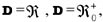, and D =Z0+.

The CaseTo decide if the sum expression Σe F x(e) is an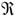-invariant of (H, q), it is sufficient to check that the incidence vector f of F in H is a linear combination of the rows hv of the incidence matrix H of H, that is, that the equation system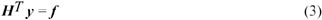is consistent (Malvestuto & Moscarini, 1990). Note that if the sum expression Σe F x(e) is an-invariant of (H, q) and y is a solution of equation system (3), then the target-query belongs to the closure of Q and the value of Q is given by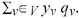It should be noted that if the sum expression Σe F x(e) is not an-invariant of (H, q), then the tightest lower and upper bounds on the value of the target-query are −∞ to +, respectively.

The Case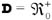One can apply linear programming methods to compute the tightest lower bound α1 and the tightest upper bound α2 on the value of the sum expression Σe F x(e), and conclude that it is an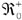-invariant of (H, q) if and only if α1 = α2. We now present an alternative method. Let kernel(H) denote the set of edges e of H such that x(e) = 0 for every solution x of the constraint system associated with Q. The set kernel(H) can be determined by solving for each edge e of H the following linear programming problem:

find the maximum value α(e) of the variable x(e) subject to the constraint system associated with Q.

Then, kernel(H) is given by the set of edges e of H for which α(e) = 0. Let K be the incidence matrix of the hypergraph K obtained from H by deleting all the edges in kernel(H), and let g be the incidence vector of the edge set Fkernel(H) in K. Then, the sum expression Σe F x(e) is an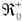-invariant of (H, q) if and only if the equation system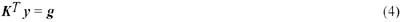is consistent (Malvestuto, 1993).

The Case D = Z0+

One can apply integer linear programming methods to compute the tightest lower bound α1 and the tightest upper bound α2 on the value of the sum expression Σe F x(e), and conclude that it is a Z0+-invariant of (H, q) if and only if α1 = α2. Note that if β1 and β2 are respectively the tightest lower and upper bounds on the value of the sum expression Σe F x(e) under the assumption D = Z0+, then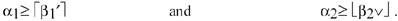It is also worth mentioning that if the incidence matrix of H is "totally unimodular" (Garfinkel & Nemhauser, 1972)—and this is the case if H is a bipartite graph—then relaxing the integrality constraints does not affect the values of the tightest lower and upper bounds on the value of the sum expression Σe F x(e), that is, α1 = β1 and α2 = β2.Multidimensional Databases: Problems and Solutions
ISBN: 1591400538
EAN: 2147483647
Year: 2003
Pages: 150